Question

Is it possible to create a buffer with NH3 and HCl as your starting materials? If your answer is no, explain why a buffer is not possible. If your answer is yes, which species would need to be in excess? Why? In either case, explain your reasoning with the use of a chemical equation.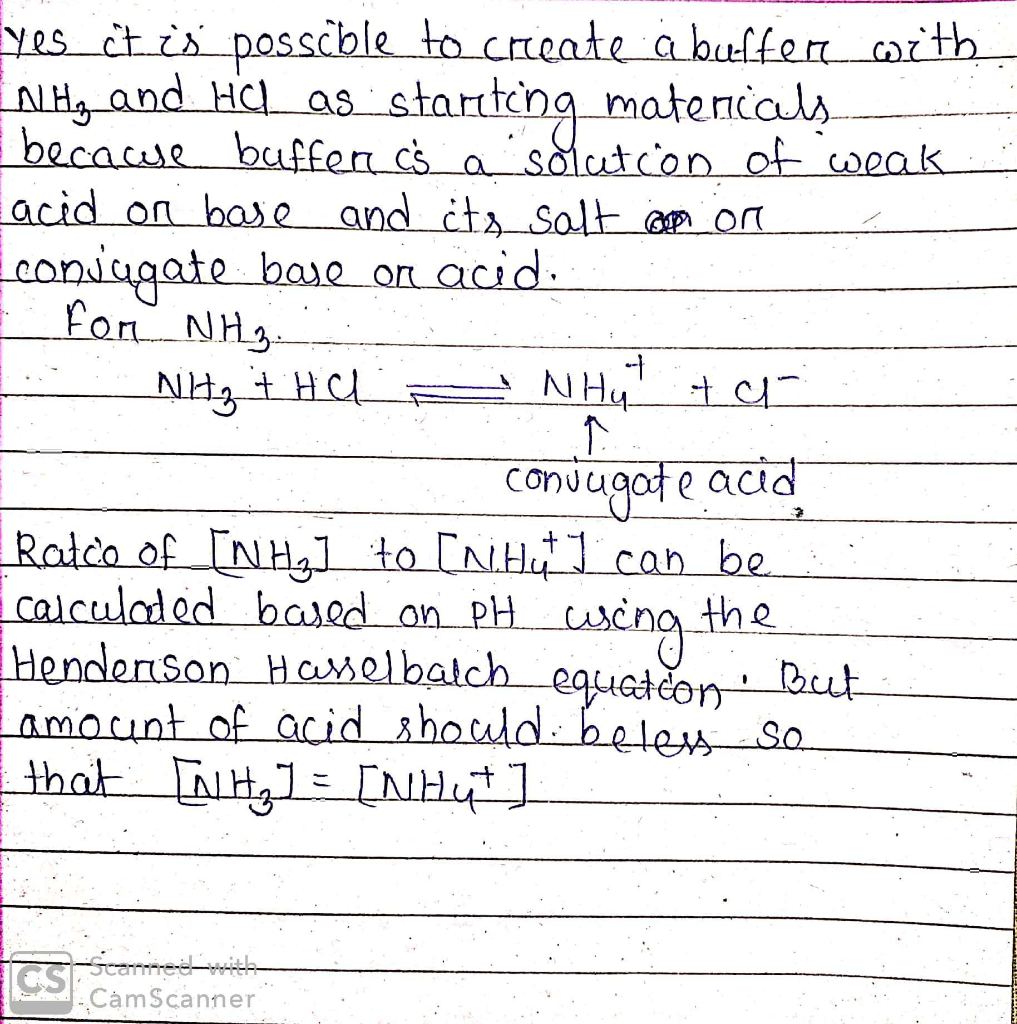#### Earn Coins

Coins can be redeemed for fabulous gifts.

Similar Homework Help Questions
• ### A buffer solution having a total volume of 0.50 L is prepared which has the following...

A buffer solution having a total volume of 0.50 L is prepared which has the following composition: [NH4Cl] = 0.25 M, [NH3] = 0.40 M. [Note: Kb of NH3 = 1.8 × 10−5 ] (a) Identify the species that acts as the acid and base in this buffer. (b) What role does the chlorine anion play? (c) Calculate the pH of this buffer solution using the Henderson-Hasselbalch equation. Is there any underlying assumption being made when you use this equation?...

• ### Conceptual questions Answer the following 5 questions. Briefly explain your reasoning in each case. (a)[3 pts]...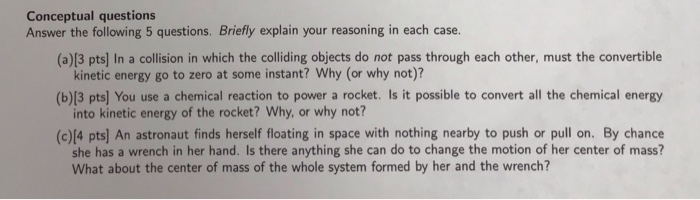Conceptual questions Answer the following 5 questions. Briefly explain your reasoning in each case. (a)[3 pts] In a collision in which the colliding objects do not pass through each other, must the convertible kinetic energy go to zero at some instant? Why (or why not)? into kinetic energy of the rocket? Why, or why not? she has a wrench in her hand. Is there anything she can do to change the motion of her center of mass? (b)[3 pts] You...

• ### Explain your answer whenever possible. Show necessary steps and reasoning For each of the following pairs...

Explain your answer whenever possible. Show necessary steps and reasoning For each of the following pairs of goods, which good would you expect to have more elastic demand and why? Refer to determinants of price elasticity of demand required textbooks or mystery novels Beethoven recordings or classical music recordings in general subway rides during the next 6 months or subway rides during the next 5 years root beer or water

• ### Show all of your work for full credit and report your final answer with the correct...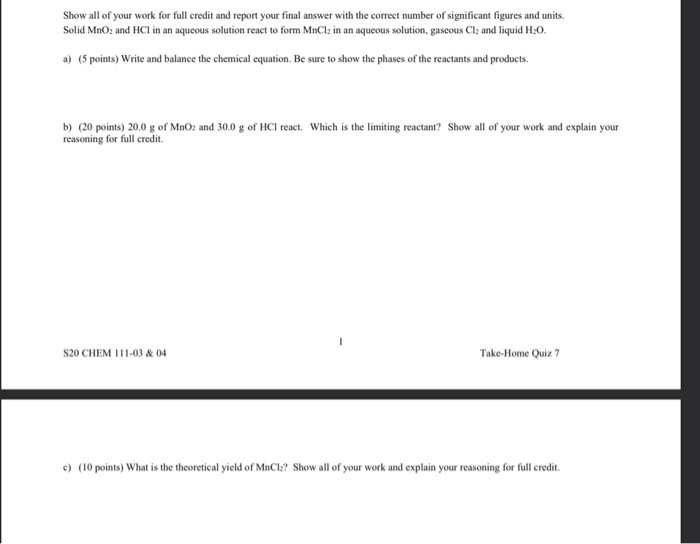Show all of your work for full credit and report your final answer with the correct number of significant figures and units. Solid MnO2 and HCl in an aqueous solution react to form MnCl2 in an aqueous solution, gaseous Clz and liquid H:O. a) (5 points) Write and balance the chemical equation. Be sure to show the phases of the reactants and products. b) (20 points) 20.0 g of MnO2 and 300 g of HCI react. Which is the limiting...

• ### Question: Answer the following question. Be as complete as possible, and always explain your reasoning. One...Question: Answer the following question. Be as complete as possible, and always explain your reasoning. One possible reaction pathway for the oxidation of 9-fluorenol with sodium hypochloride solution and acetic acid proceeds through a reaction intermediate A (not isolated). Intermediate A then reacts further to provide the desired product Draw the chemical structures of reaction intermediate A and product B. Write a reasonable mechanism for the conversion of intermediate A to 9-fluorenone. Be as complete as possible and show electron...

• ### your exam. What is the wavelength of a wave (in cm) with a frequency of 3.45...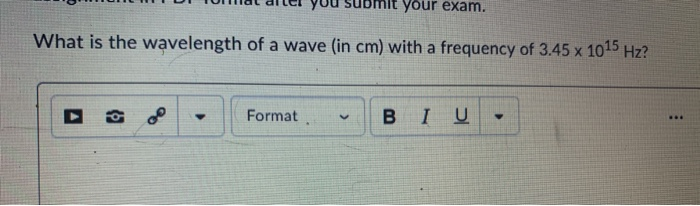your exam. What is the wavelength of a wave (in cm) with a frequency of 3.45 x 1015 Hz? Format BI U . Draw Dot Structures for both SeF4 and PCls. Explain differences in polarity (if any) and why they are different or the same. 4 Question 42 (6 points) On the basis of bonding principles, predict whether the following compounds exist. . Blank #1: NF5 (yes or no) • Blank #2: Explain your reasoning for your answer in Blank...

• ### pH of 9.2 using ammonium nitrate: pKa= 9.24 2 Introduiction The Henderson-Hasselbalch equation, or A-1 HAl relates the pH of a buffer with the pKo of the acid and the concentration of the conju...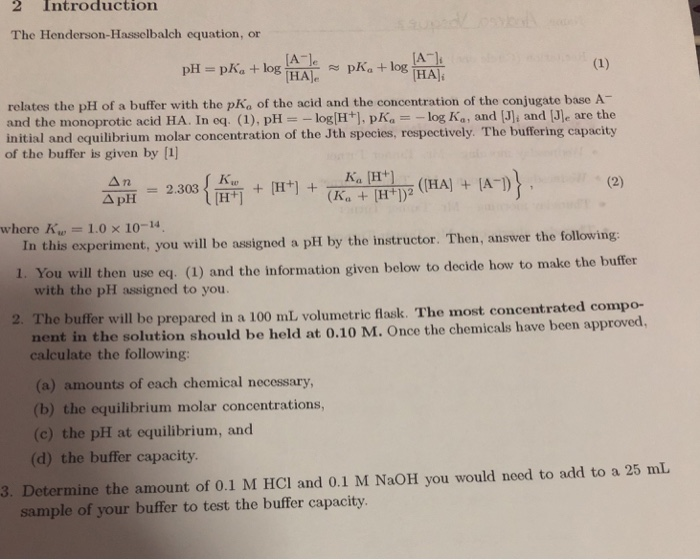pH of 9.2 using ammonium nitrate: pKa= 9.24 2 Introduiction The Henderson-Hasselbalch equation, or A-1 HAl relates the pH of a buffer with the pKo of the acid and the concentration of the conjugate base A- and the monoprotic acid HA. In cq. (1), pH-_ log[H+], pK, =-log Ka, and [J]i and [JIe are the initial and equilibrium molar concentration of the Jth species, respectively. The buffering capacity of the buffer is given by  Ka H+ where K,e-1.0 ×...

• ### please answer number 5 and 6 5. The buffer range for a given weak acid/conjugate base pair is pK, 11. Explain why. 6...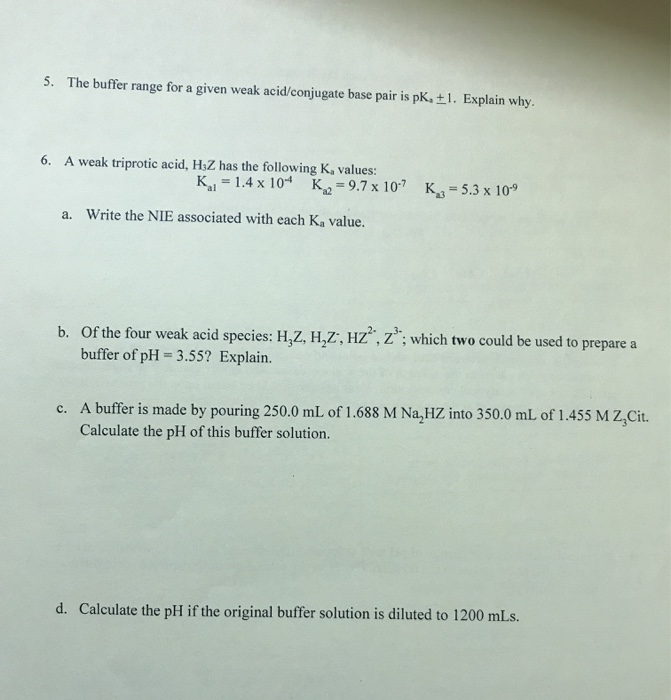please answer number 5 and 6 5. The buffer range for a given weak acid/conjugate base pair is pK, 11. Explain why. 6. A weak triprotic acid, HZZ has the following K, values: Ki = 1.4 x 104 K = 9.7 x 10-7 K 5.3 x 109 a. Write the NIE associated with each Ka value. b. Of the four weak acid species: H.Z, H,Z, HZz"; which two could be used to prepare a buffer of pH = 3.55? Explain....

• ### please solve 4 of them, thank you. 2. Buffer Questions (Do 4 of 5 problems---6 pts...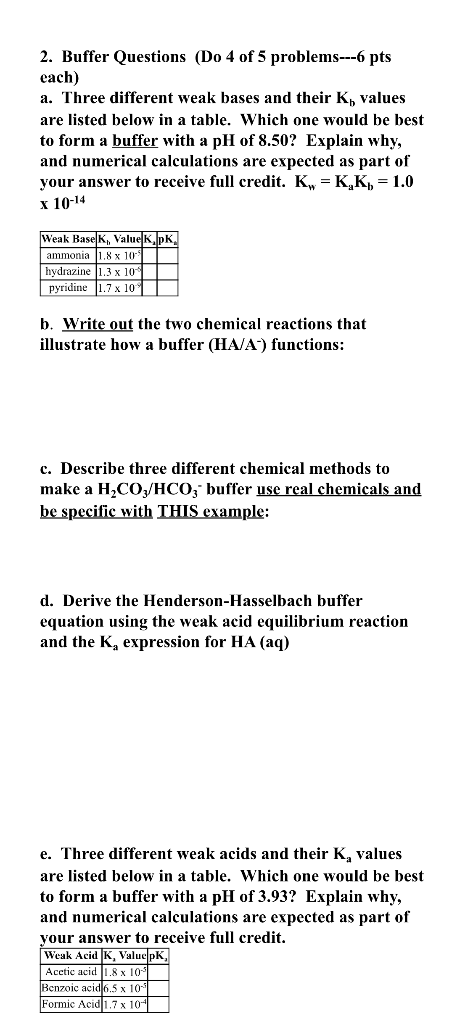please solve 4 of them, thank you. 2. Buffer Questions (Do 4 of 5 problems---6 pts each) a. Three different weak bases and their Kh values are listed below in a table. Which one would be best to form a buffer with a pH of 8.50? Explain why, and numerical calculations are expected as part of your answer to receive full credit. K = K,K) = 1.0 x 10-14 Weak Base K, Value KPK ammonia (1,8 x 10 hydrazine 1.3...